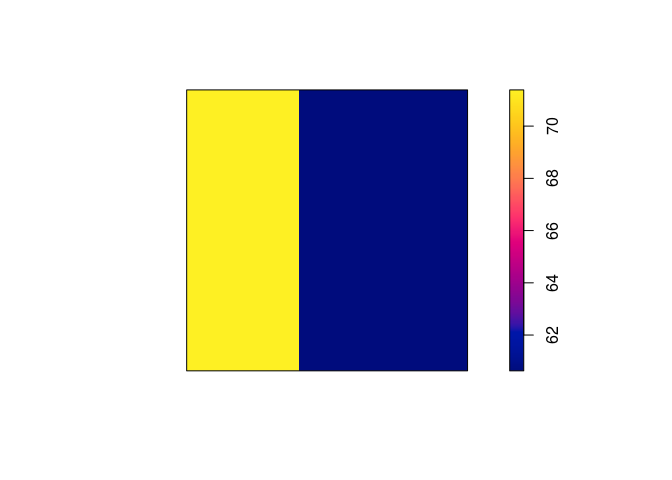# Lab 4: Fitting Poisson models

This session is concerned with Poisson point process models. The lecturer’s R script is available here (right click and save).

``````library(spatstat)
``````

### Exercise 1

The command `rpoispp(100)` generates realisations of the Poisson process with intensity λ = 100 in the unit square.

1. Repeat the command `plot(rpoispp(100))` several times to build your intuition about the appearance of a completely random pattern of points.

Let’s plot it three times:

``````replicate(3, plot(rpoispp(lambda = 100), main = ""))
``````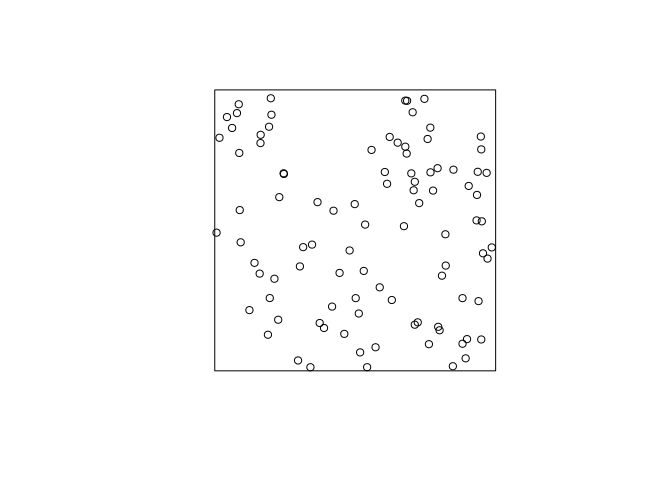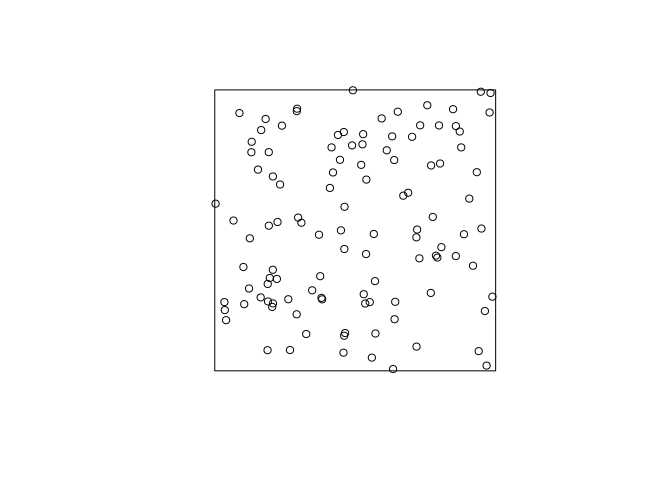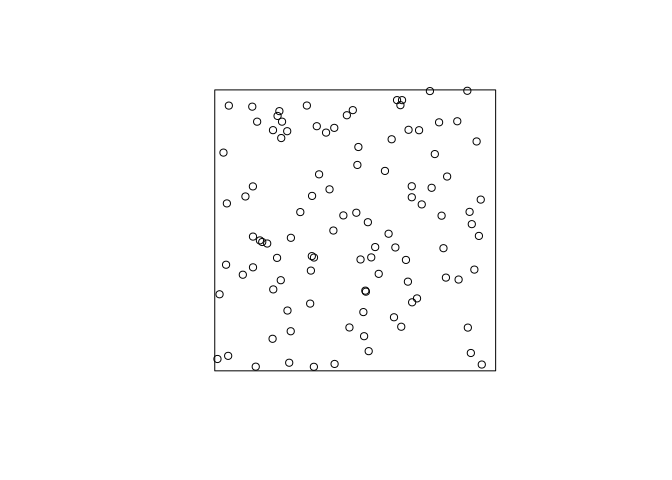As can be seen, the points (unsurprisingly) are much more random that want one might think. “Randomly” drawing points on a piece of paper one would usually draw a point pattern that is more regular (i.e. the points are repulsive).

2. Try the same thing with intensity λ = 1.5.

For brevity we only do it once here:

``````plot(rpoispp(lambda = 1.5), main = "")
``````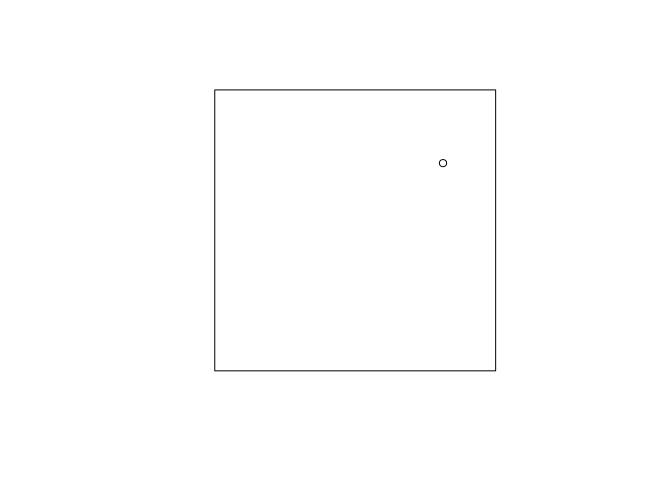Here we expect 1.5 points in the plot each time.

### Exercise 2

Returning to the Japanese Pines data,

1. Fit the uniform Poisson point process model to the Japanese Pines data

``````ppm(japanesepines~1)
``````

We fit the Poisson process model with the given command and print the output:

``````m.jp <- ppm(japanesepines ~ 1)
print(m.jp)
``````
``````## Stationary Poisson process
## Intensity: 65
##             Estimate      S.E.  CI95.lo  CI95.hi Ztest     Zval
## log(lambda) 4.174387 0.1240347 3.931284 4.417491   *** 33.65499
``````
2. Read off the fitted intensity. Check that this is the correct value of the maximum likelihood estimate of the intensity.

We extract the coeficient with the `coef` function, and compare to the straightforward estimate obtained by `intensity``:

``````unname(exp(coef(m.jp)))
``````
``````##  65
``````
``````intensity(japanesepines)
``````
``````##  65
``````

As seen, they agree exactly.

### Exercise 3

The `japanesepines` dataset is believed to exhibit spatial inhomogeneity.

1. Plot a kernel smoothed intensity estimate.

Plot the kernel smoothed intensity estimate selecting the bandwidth with `bw.scott`:

``````jp.dens <- density(japanesepines, sigma = bw.scott)
plot(jp.dens)
plot(japanesepines, col = "white", cex = .4, pch = 16, add = TRUE)
``````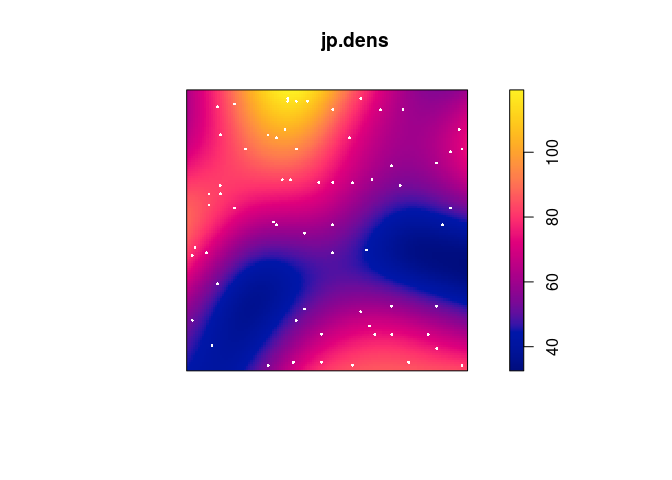2. Fit the Poisson point process models with loglinear intensity (trend formula `~x+y`) and log-quadratic intensity (trend formula `~polynom(x,y,2)`) to the Japanese Pines data.

We fit the two models with `ppm`:

``````jp.m <- ppm(japanesepines ~ x + y)
jp.m2 <- ppm(japanesepines ~ polynom(x, y, 2) )
``````
3. extract the fitted coefficients for these models using `coef`.

``````coef(jp.m)
``````
``````## (Intercept)           x           y
##   4.0670790  -0.2349641   0.4296171
``````
``````coef(jp.m2)
``````
``````## (Intercept)           x           y      I(x^2)    I(x * y)      I(y^2)
##   4.0645501   1.1436854  -1.5613621  -0.7490094  -1.2009245   2.5061569
``````
4. Plot the fitted model intensity (using `plot(predict(fit))`)

``````par(mar=rep(0,4))
plot(predict(jp.m), main = "")
``````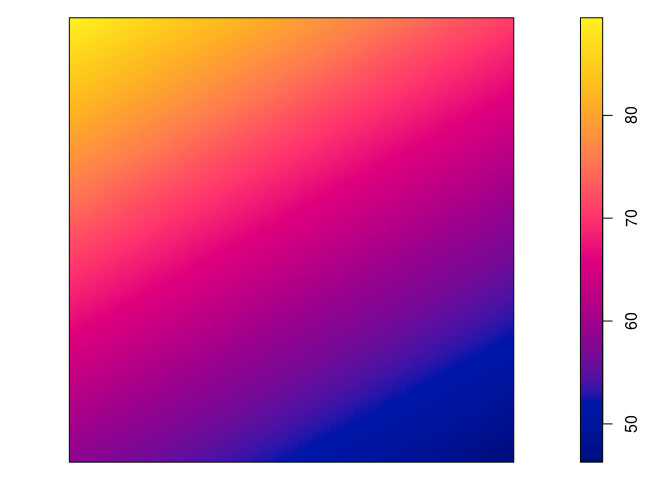``````plot(predict(jp.m, se=TRUE)\$se, main = "")
``````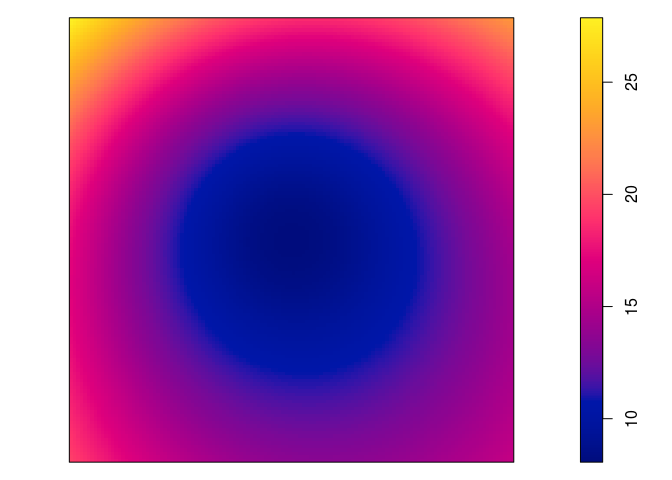``````plot(predict(jp.m2), main = "")
``````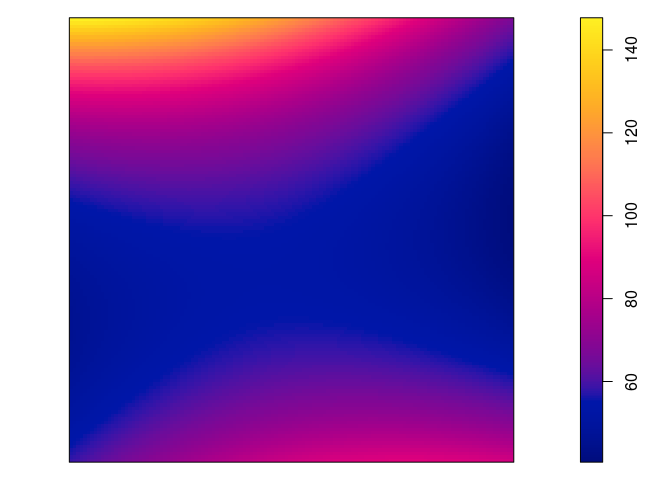``````plot(predict(jp.m2, se=TRUE)\$se, main = "")
``````5. perform the Likelihood Ratio Test for the null hypothesis of a loglinear intensity against the alternative of a log-quadratic intensity, using `anova`.

``````anova(jp.m, jp.m2)
``````
``````## Analysis of Deviance Table
##
## Model 1: ~x + y   Poisson
## Model 2: ~x + y + I(x^2) + I(x * y) + I(y^2)      Poisson
##   Npar Df Deviance
## 1    3
## 2    6  3   3.3851
``````
6. Generate 10 simulated realisations of the fitted log-quadratic model, and plot them, using `plot(simulate(fit, nsim=10))` where `fit` is the fitted model.

``````par(mar=rep(0.5,4))
plot(simulate(jp.m2, nsim=10), main = "")
``````
``````## Generating 10 simulated patterns ...1, 2, 3, 4, 5, 6, 7, 8, 9,  10.
``````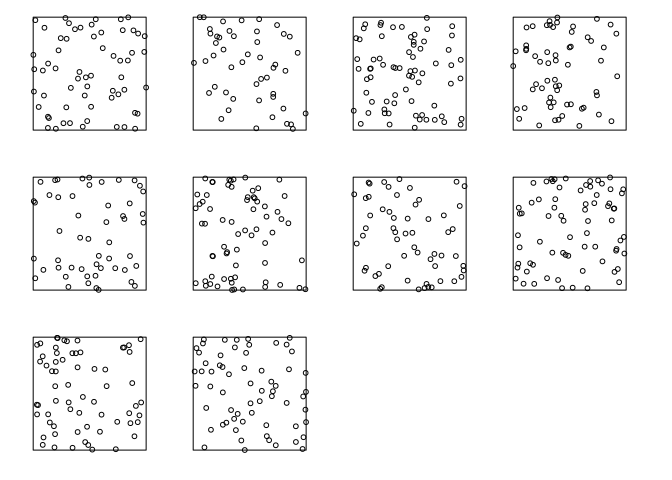### Exercise 4

The `update` command can be used to re-fit a point process model using a different model formula.

1. Type the following commands and interpret the results:

``````fit0 <- ppm(japanesepines ~ 1)
fit1 <- update(fit0, . ~ x)
fit1
``````
``````## Nonstationary Poisson process
##
##
## Fitted trend coefficients:
## (Intercept)           x
##   4.2895587  -0.2349362
##
##               Estimate      S.E.   CI95.lo   CI95.hi Ztest       Zval
## (Intercept)  4.2895587 0.2411952  3.816825 4.7622926   *** 17.7845936
## x           -0.2349362 0.4305416 -1.078782 0.6089098       -0.5456759
``````
``````fit2 <- update(fit1, . ~ . + y)
fit2
``````
``````## Nonstationary Poisson process
##
##
## Fitted trend coefficients:
## (Intercept)           x           y
##   4.0670790  -0.2349641   0.4296171
##
##               Estimate      S.E.    CI95.lo   CI95.hi Ztest       Zval
## (Intercept)  4.0670790 0.3341802  3.4120978 4.7220602   *** 12.1703167
## x           -0.2349641 0.4305456 -1.0788181 0.6088898       -0.5457357
## y            0.4296171 0.4318102 -0.4167154 1.2759495        0.9949211
``````

OK, let’s do that:

``````fit0 <- ppm(japanesepines ~ 1)
fit1 <- update(fit0, . ~ x)
fit1
``````
``````## Nonstationary Poisson process
##
##
## Fitted trend coefficients:
## (Intercept)           x
##   4.2895587  -0.2349362
##
##               Estimate      S.E.   CI95.lo   CI95.hi Ztest       Zval
## (Intercept)  4.2895587 0.2411952  3.816825 4.7622926   *** 17.7845936
## x           -0.2349362 0.4305416 -1.078782 0.6089098       -0.5456759
``````
``````fit2 <- update(fit1, . ~ . + y)
fit2
``````
``````## Nonstationary Poisson process
##
##
## Fitted trend coefficients:
## (Intercept)           x           y
##   4.0670790  -0.2349641   0.4296171
##
##               Estimate      S.E.    CI95.lo   CI95.hi Ztest       Zval
## (Intercept)  4.0670790 0.3341802  3.4120978 4.7220602   *** 12.1703167
## x           -0.2349641 0.4305456 -1.0788181 0.6088898       -0.5457357
## y            0.4296171 0.4318102 -0.4167154 1.2759495        0.9949211
``````
2. Now type `step(fit2)` and interpret the results.

The backwards selection is done with the code:

``````step(fit2)
``````
``````## Start:  AIC=-407.96
## ~x + y
##
##        Df     AIC
## - x     1 -409.66
## - y     1 -408.97
## <none>    -407.96
##
## Step:  AIC=-409.66
## ~y
##
##        Df     AIC
## - y     1 -410.67
## <none>    -409.66
##
## Step:  AIC=-410.67
## ~1

## Stationary Poisson process
## Intensity: 65
##             Estimate      S.E.  CI95.lo  CI95.hi Ztest     Zval
## log(lambda) 4.174387 0.1240347 3.931284 4.417491   *** 33.65499
``````

First, given two models the preferred model is the one with the minimum AIC value. In step 1, the removal of x results in the least AIC and is hence deleted. In step 2, removing y results in a lower AIC than not deleing anything and is thus deleted. This results in the constant model.

### Exercise 5

The `bei` dataset gives the locations of trees in a survey area with additional covariate information in a list `bei.extra`.

1. Fit a Poisson point process model to the data which assumes that the intensity is a loglinear function of terrain slope and elevation (hint: use `data = bei.extra` in `ppm`).

We fit the log-linear intensity model with the following:

``````bei.m <- ppm(bei ~ elev + grad, data = bei.extra)
``````
2. Read off the fitted coefficients and write down the fitted intensity function.

The coefficents are extraced with `coef`:

``````coef(bei.m)
``````
``````## (Intercept)        elev        grad
## -8.55862210  0.02140987  5.84104065
``````

Hence the model is log**λ(u)= − 8.55 + 0.02 ⋅ E(u)+5.84G(u) where E(u) and G(u) is the elevation and gradient, respectively, at u.

3. Plot the fitted intensity as a colour image.

``````plot(predict(bei.m), main = "")
plot(bei, cex = 0.3, pch = 16, cols = "white", add = TRUE)
``````4. extract the estimated variance-covariance matrix of the coefficient estimates, using `vcov`.

We call `vcov` on the fitted model object:

``````vcov(bei.m)
``````
``````##               (Intercept)          elev          grad
## (Intercept)  0.1163496911 -7.774152e-04 -0.0354946468
## elev        -0.0007774152  5.233903e-06  0.0001993179
``````
5. Compute and plot the standard error of the intensity estimate (see `help(predict.ppm)`).

From the documentation the argument `se` will trigger the computation of the standard errors. These are then plotted in the standard manner.

``````std.err <- predict(bei.m, se = TRUE)\$se
plot(std.err, main = "")
``````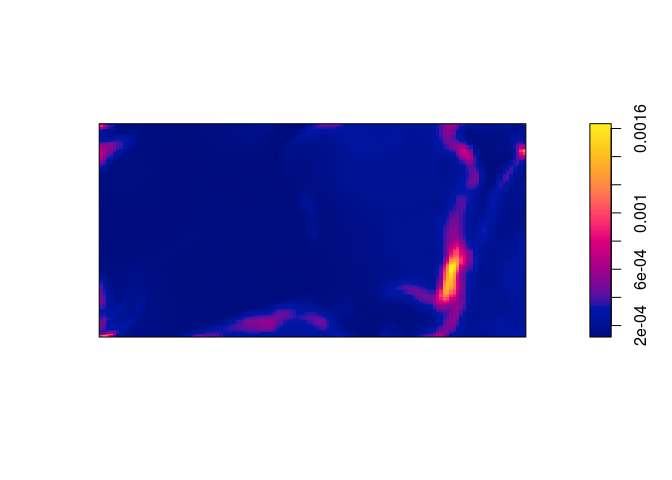### Exercise 6

Fit Poisson point process models to the Japanese Pines data, with the following trend formulas. Read off an expression for the fitted intensity function in each case.

Trend formula Fitted intensity function
`~1` logλ(u)=4.17
`~x` logλ(u)=4.28 − 0.23x
`~sin(x)` logλ(u)=4.29 − 0.26sin(x)
`~x+y` logλ(u)=4.07 − 0.23x + 0.42y
`~polynom(x,y,2)` logλ(u)=4.06 + 1.14x − 1.56y − 0.75x2 − 1.20xy + 2.51y2
`~factor(x < 0.4)` logλ(u)=4.10 + 0.16 ⋅ I(x < 0.4)

(Here, I(⋅) denote the indicator function.)

The fitted intensity functions have been written into the table based on the follwing model fits:

``````coef(ppm1 <- ppm(japanesepines ~ 1))
``````
``````## log(lambda)
##    4.174387
``````
``````coef(ppm2 <- ppm(japanesepines ~ x))
``````
``````## (Intercept)           x
##   4.2895587  -0.2349362
``````
``````coef(ppm3 <- ppm(japanesepines ~ sin(x)))
``````
``````## (Intercept)      sin(x)
##   4.2915935  -0.2594537
``````
``````coef(ppm4 <- ppm(japanesepines ~ x + y))
``````
``````## (Intercept)           x           y
##   4.0670790  -0.2349641   0.4296171
``````
``````coef(ppm5 <- ppm(japanesepines ~ polynom(x, y, 2)))
``````
``````## (Intercept)           x           y      I(x^2)    I(x * y)      I(y^2)
##   4.0645501   1.1436854  -1.5613621  -0.7490094  -1.2009245   2.5061569
``````
``````coef(ppm6 <- ppm(japanesepines ~ factor(x < 0.4)))
``````
``````##         (Intercept) factor(x < 0.4)TRUE
##           4.1048159           0.1632665
``````

### Exercise 7

Make image plots of the fitted intensities for the inhomogeneous models above.

Again, we use `plot(predict())`:

``````plot(predict(ppm1), main = "")
````````````plot(predict(ppm2), main = "")
``````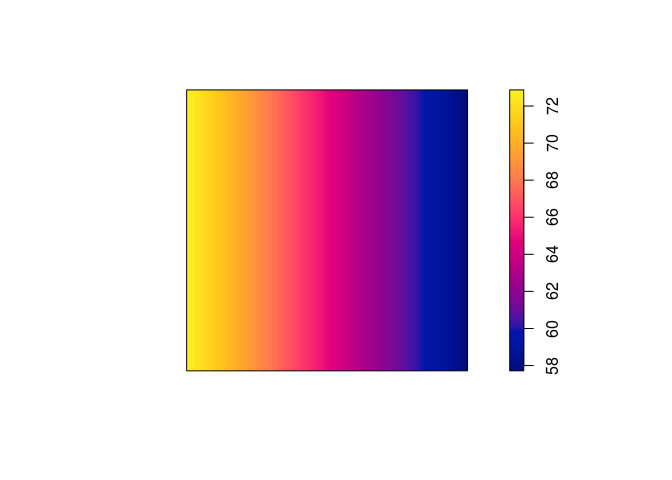``````plot(predict(ppm3), main = "")
``````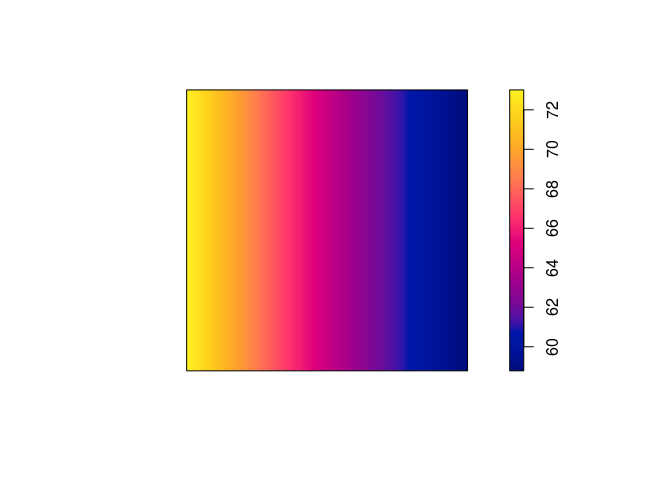``````plot(predict(ppm4), main = "")
````````````plot(predict(ppm5), main = "")
``````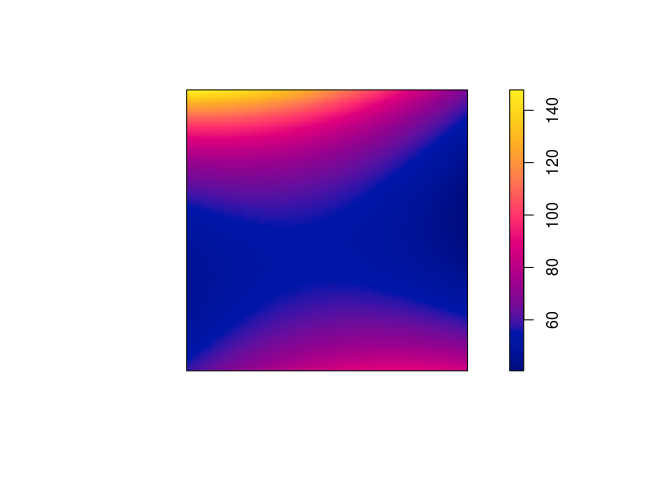``````plot(predict(ppm6), main = "")
``````